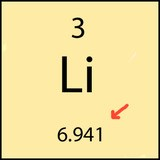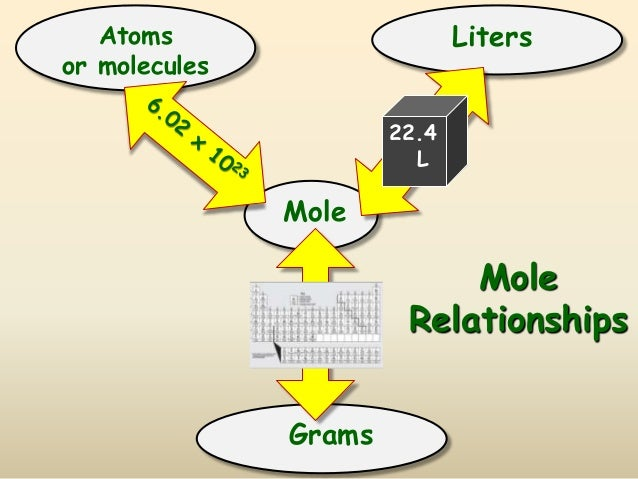# The Mole

The content that follows is the substance of lecture 8. In this lecture we cover the Mole and Avagadro's Number as well as the calculations for Molar Mass and conversions using moles.

The MOLE (mol) is a unit of measurement that is the amount of a pure substance containing the same number of chemical units (atoms, molecules etc.) as there are atoms in exactly 12 grams of carbon-12 (i.e., 6.022 X 1023).

So the mole is the title used for the amount 6.022 x 1023 much the same way the word "dozen" is used for the amount 12.

So if you had a mole of donuts you would have 6.022 x 1023 donuts and a serious stomach ache.

We use the mole (mol) to represent the amount of substances in chemistry because the numbers of atoms and molecules in each substance is so large. The value given 6.022 x 1023 is called Avagadro's number for the scientist that found the number of atoms in 12 grams of carbon 12. Why use 12 grams? This is the theoretical atomic mass of the Carbon-12 isotope (6 protons and 6 neutrons). This means that the atomic mass or atomic weight (12 grams) of carbon is equal to exactly 1 mole of carbon.

Using carbon as a reference, the atomic weights you see in the periodic table are also equal to one mole of those substances:Lithium for instance has an atomic mass of 6.941 grams and this is equal to one mole of lithium. This is why we state the atomic and molecular masses in units of grams per mole or g/mol.

What can we do with moles? We use the unit to make calculations based on balanced chemical equations. We use the stoichiometry (fancy way of saying mole ratios in an equation) to make predictions about how much product will be made or reactant needed if we know one mole amount in a reaction.

### Moles of a Substance and the Molecular Weight

The Molar mass or Molecular Weight (interchangeable terms so long as we are on Earth) of a substance is the total of all the individual masses of the elements it contains. To use our old friend water as an example:

One mole of Water is composed of 1 mole of Oxygen and two moles of Hydrogen. The mass of oxygen equal to one mole of oxygen is 15.998 grams and the mass of one mole of hydrogen is 1.008 g. If we total up the gram amounts of each element in the water molecule = 15.998g/mol + 2(1.008g/mol) we get the molar mass of water = 18.014g/mol.

So if we had exactly 18.014g of water we would have 1 mole of water.

### Mole RelationshipsThe reason the mole is so important is because we use the mole as the unit for most of the relationships in chemistry. Reactions are balanced based on the number of moles of each element in the reaction, solution concentrations are very often described in terms of moles per liter or moles per kg of solvent and we have already seen that the molecules or atoms of an element are reported as moles of the substance rather than the individual count of their particles.

Let's start the relationship discussion with the relationship between the mole and the AMU.

An AMU is 1/12 the mass of a Carbon-12 atom which according to the periodic table weighs ~12g. This means that 1 AMU ≈ 1g right? and thus Carbon has a mass of 12amu, correct? But wait, this could not be the mass of a single carbon atom right? They are really, really tiny. And that is correct, the mass shown in the periodic table is actually the mass of 6.022 x 1023 carbon atoms or a mole of carbon atoms. So in reality, 1 AMU = 1.66 x 10-24g and while a carbon atom weighs 12 AMU, the mass shown in the periodic table is

### 12 atoms x 1.66 x 10-24g x 6.022 x 1023 atoms/ mol= 12 g/mol

So a single carbon atom weighs 12 amu while a mole of carbon atoms weighs 12.01g/mol.

## Mole Calculations

There are plethora of calculations that can be conducted using the unit of moles as the intermediate. I am going to review a few of them now and then you will see as we progress through later topics that moles are essential to those calculations as well.

Typical mole calculations:

1) Calculation of the Molecular Weight or Molar Mass of a compound

The molar mass of a compound also often called the molecular weight while on Earth, is simply the sum of all the individual element masses in the compound. You use the periodic table as a reference for these masses and total them up:

For example, if we want to total the molar mass of Aluminum Sulfate Al2(SO4)3 , we need to determine the number and mass of each element in the compound. For Aluminum Sulfate the totals are 2 Al, 3 S and 12 O. So here is the math using the atomic masses given in the periodic table:

### 2(26.98 g/mol) + 3( 32.07 g/mol) + 12(16.00 g/mol) = 342.17 g/mol

2) Conversion from Grams to Moles or Moles to Grams

Once you know the molar mass of a compound, you can use that mass to determine the amount of moles in a gram amount of the substance or conversely, you can calculate the number of grams in a mole amount of the substance.

Here are some examples:

How many moles are in 55.4g of Aluminum Sulfate?

The calculation is set up like a conversion with the molar mass of Aluminum Sulfate as the conversion factor:

### 55.4g Al2(SO4)3 x 1 mol Al2(SO4)3/342.17 g Al2(SO4)3 = 0.162 mol Al2(SO4)3

Or, How many grams of Al2(SO4)3 are in 6.34 moles of Al2(SO4)3?

### 6.34 mol Al2(SO4)3 x 342.17g Al2(SO4)3/ 1 mol Al2(SO4)3 = 2.17 x 103 Al2(SO4)3

3) Conversion to Molecules or Atoms

In addition to being a unit for molar mass, the mole is also the gateway between the mass of a substance and its atoms or molecules.

For Example, if asked how many molecules of Al2(SO4)3 are in th 55.4 g of Al2(SO4)3 above we simply need to first calculate the number of moles as before and then use Avagadro's number to convert the moles to molecules:

### 9.76 x 1022 Molecules Al2(SO4)3

Furthermore if we continued the problem and asked how many Aluminum atoms were in the 55.4 g of Al2(SO4)3?

Well that is a simple continuation. We just need to multiply by the number of atoms of Aluminum in the compound:

### 9.76 x 1022 Molecules Al2(SO4)3 x 2 atoms Al/ 1 molecule Al2(SO4)3 = 1.95 x 1023 Al atoms

Let's Practice some more: